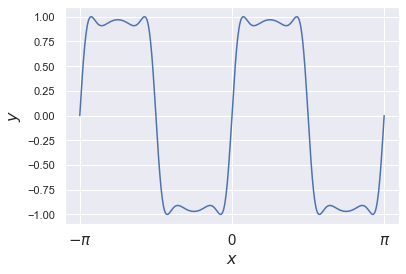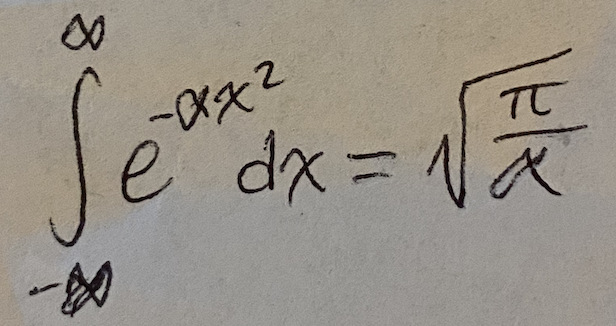# Tutorial 0b: Using Jupyter Notebooks

## What is a Jupyter notebook?

Jupyter is browser-based system to write code, math, and text in the same document such that you can clearly explain the concepts and practices used you your program. Jupyter is not only for Python, but can be used with R, Juila, MATLAB, and about 35 other languages as of this writing. All files are saved as a JSON formatted text file with the extension .ipynb. For homework in this class, you will send this text file via email to the TAs.

This tutorial assumes that you have followed Tutorial 0a: Setting Up Python, which covers how to launch a Jupyter Notebook from JupyterLab.

## Writing code

All code you write in the notebook will be in the code cell. You can write single lines, to entire loops, to complete functions. As an example, we can write and evaluate a print statement in a code cell, as is shown below. To exectue the code, we can simply hit shift + enter while our cursor is in the code cell.

# This is a comment and is not read by Python
print('Hello! This is the print function. Python will print this line below')

Hello! This is the print function. Python will print this line below


The box with the gray background contains the python code while the output is in the box with the white background. We can also write a for loop as an example of executing multiple lines of code at once.

# Write a basic for loop.
for i in range(5):
# Multiply the value of i by two and assign it to a variable.
temp_variable = 2 * i

# Print the value of the temp variable.
print(temp_variable)

0
2
4
6
8


As is discussed in the Python syntax tutorial, we often must import modules to get scientific power out of our programs. Import statements work in these cells as well. For example, we can import multiple modules at once that we will use to make a plot.

import numpy as np
import matplotlib.pyplot as plt
import seaborn as sns
sns.set() # This line sets the style through seaborn to make the plot look nicer.


As an example, we can plot again the same function as in the setup tutorial.

# Plot one of Griffin's favorite functions.
x = np.linspace(-np.pi, np.pi, 500)
y = np.sin(2 * np.sin(2 * np.sin(2 * x)))

# Generate the plot.
plt.plot(x, y)
plt.xlabel(r'$x$', fontsize=16)
plt.ylabel(r'$y$', fontsize=16)

_ = plt.xticks([-np.pi, 0, np.pi], ['$-\pi$', '0', '$\pi$'], fontsize=15)While you could in principle write an entire script’s worth of code in a single code cell, this is not wise for readability. Therefore, keep the length of what you write in code cells to a logical minimum. Write them such that the perform a single step in your analysis, then move on to the next cell. For example, it is wise to write a function in its own code cell and then execute it in another. It is reasonable, however, to have a single notebook contain all code for a single analysis, just not in a single code cell.

### A warning

Code cells can be executed in any order. This means that you can overwrite your current variables by running things out of order. When coding in notebooks, be cautions of the order in which you run cells.

## Writing Text

Arguably the most useful component of the Jupyter notebook is the ability to interweave code and explanatory text into a single, coherent document. For the purposes of this course, all code and plots should be accompanied with explanatory text. The only way for those of us reading your work to understand your thought process depends on your explanation.

Each cell in a Jupyter notebook can exist either as a code cell or as text-formatting cell called a markdown cell. Markdown is a mark-up language that very easily converts to other typesetting formats such as HTML and PDF. You can find a cheat sheet here.

Whenever you make a new cell, it’s default assignment will be a code cell. This means when you want to write text, you will need to specifically change it to a markdown cell. You can do this by clicking on the drop-down menu that reads code and selecting ‘Markdown’. You can then type in the code cell and all Python syntax highlighting will be removed. The basics of Markdown (nearly all you will need to know can be summarized by typing the following lines into a markdown cell.

**This part of the sentence will be bold**, *this part italic*, [this part a link](http://www.google.com), and
* This part a
+ bulleted
- list


These lines yield the following output:

This part of the sentence will be bold, this part italic, this part a link, and

• This part a
• bulleted
• list

Mathematics can be typeset using the LaTeX typesetting language within markdown cells. For example, typing

$$\int\limits_{-\infty}^{\infty}e^{-\alpha x^2} dx = {\sqrt{\pi}\over{\alpha}}$$


into a markdown cell is rendered as

which is the infamous gaussian integral. Typsetting math in this manner is not required for the course. If mastering LaTeX is too challenging, you may include highly legible scans of your hand-written math in the following way.

![](path/to/image/gaussian_integral.jpg)


The above line yields the following image.Note that this image must also be included in your homework submission email.

## Saving, quitting, and going home

Jupyter notebooks are set up to autosave your work every 15 or so minutes. However, you should not rely on the autosave feature! Save your work frequently by clicking on the floppy disk icon located in the upper left-hand corner of the toolbar.

To navigate back to the root of your Jupyter notebook server, you can click on the Jupyter logo at any time.

To quit your Jupyter notebook, you can simply close the browser window and Anaconda Navigator.

## Converting to HTML and PDF

For this course, you must submit your coding submissions in the .ipynb format. However, it is useful to know that you can convert these notebooks to highly-portable formats such as HTML and PDF. To convert, you can either do this using the dropdown menu option File -> download as -> ... or via the command line by using the following lines:

jupyter nbconvert notebook_name.ipynb notebook_name.pdf


assuming you are in the same working directory as your notebooks.